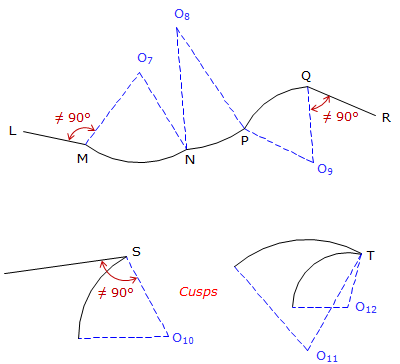## 1. Arcs

Many parcel boundaries contain curved lines. Geometric curved lines are generally sections of circular arcs since those are the most prevalent in horizontal alignments. Knowing something about the geometry of a circular arc, and its relationship to adjacent lines, makes it easier to deal with them correctly when reducing survey data.

## 2. Geometry

### a. Nomenclature; Geometric elements

 Δ: Central angle; also the deflection angle between the tangents. R: Radius L: Arc length C: Chord length T: Tangebt distanceFigure F-1 Parts and NomenclatureEquation F-1 Equation F-2 Equation F-3

When dealing with highway or railroad alignments, another geometric element is often specified: degree of curvature, D. There are two different degree of curvature definitions:

DA, arc definition; the angle subtended by a 100.00' arc, Figure F-2(a).

DC, chord definition; the angle subtended by a 100.00' chord, Figure F-2(b).(a) Arc Definition (b) Chord Definition Figure F-2 Degree of Curvature

Arc definition is used in highway and street design while the Chord definition is used in railroad design. Because each definition differs, there is a slightly different radius relationship for each:Equation F-4 Equation F-5 R: Radius (ft) D: Degree of curvature (DMS)      DA: Arc Definition      DC: Chord Definition

Degree of curvature is an exclusively English units system attribute as there is no 100.00' equivalent in the metric system. Because of this, we won't use it here in the COGO section.

### b. Sectors; Segments

Sectors and segments are parts of a circle and used to compute areas enclosed by curvilinear boundaries.Figure F-3 Figure F-4Equation F-6 Equation F-7

## 3. Tangency Condition

At its ends, an arc can be joined to a straight line or another arc. At the connection point, the relationship with the adjacent boundary can be tangent or non tangent.

For a tangent condition, Figure F-5,

Line to arc (and arc to line) - line is perpendicular to the radius at the intersection.

Arc to Arc - intersection point and radius point of both arcs are collinear; all three point are on a straight line.Figure F-5 Tangent Conditions

Any deviation from these constraints results in a non-tanget transition, Figure F-6.Figure F-6 Nontangent Conditions

## 4. Curvilinear Traverse

A curvilinear traverse is one which includes at least one arc as a side.Figure F-7 Curvilinear Traverse

Some of the standard traverse computations need modification in order to account for curves. For example, latitudes and departures are determined for straight lines; on an arc this would correspond to the its chord. Another issue concerns area computation which we'll examine in a bit.

To fix the geometry and orientation of an arc, we need at least two of its geometric elements (e.g., R, Δ, L, T, C) along with its tangency conditions to adjacent elements. Given sufficient arc data we can use COGO tools in conjunction with arc geometry to compute point positions. This gives us the ability to compute more complex collections of traverses such as Figure F-8 (taken from the Wisconsin Platting Manual, Plat Review, Wisconson Dept of Administration).Figure F-8 Multiple Curvilinear Traverses
Hits: 8895